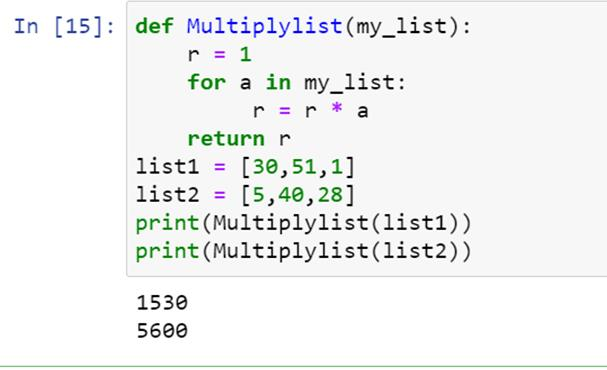"
Last Updated: Thursday 30th December 2021

Multiplication is a fundamental operation in the arithmetic and programming world. We can find its use in every program(or in the logic behind every code), no matter how basic it is. Thus a programmer must know how to multiply integers, decimals, complex numbers, and strings in Python to create an efficient and accurate code. In this article, we will learn how to perform all these operations with coding examples.

But first, let’s see what the syntax is to denote multiplication operation. We use the asterisk character ‘*’ to perform multiplication. Let’s consider an example, suppose we have to multiply two numbers, 171 and 899. Then the code for this operation is :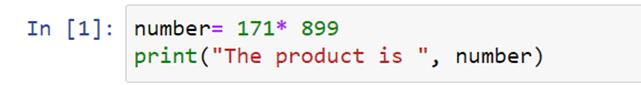The output for the code mentioned above is :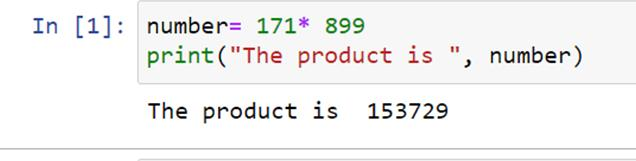We can see that we use the asterisk operator for writing multiplication codes in Python.

## Multiplying Integers In Python

Integers are a data type consisting of only whole numbers, i.e., there are no fractional parts in integers. For example 117, 1, 2 ,10 are integers. The syntax for multiplying integers is straightforward. We write the numbers to be multiplied and separate them by the asterisk operator.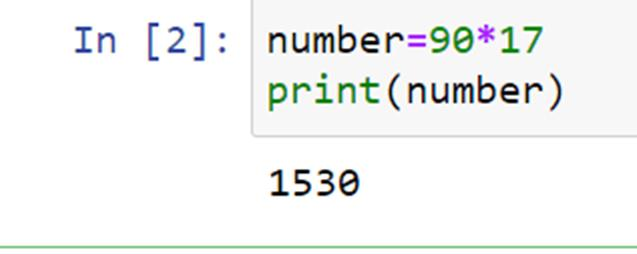We can see the multiplication of the numbers 90 and 17 in the complete code snippet given above.

## Multiplying Float Numbers In Python

The basic definition of the float number data type is that it contains numbers comprising of fractions. It can store numbers having up to seventeen significant digits. Examples of float numbers are 17.003, 5.0098, 70.0007, and many more such numbers.

The syntax for multiplying float numbers is the same as that of integers; we separate the numbers we have to multiply by the asterisk operator and print it.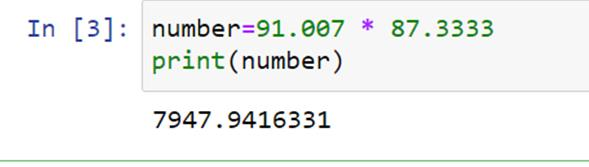In this code snippet, we have multiplied two float numbers, 91.007 and 87.3333, using the asterisk operator and printed it. We can note here that the output of the multiplication is also a float number.

## Multiplying Complex Numbers In Python

Complex numbers are imaginary numbers of the form ‘a+ bi’, where 'a' represents the real number and 'b' is the coefficient of the imaginary number. Also,' i' represents 'iota,' which is the square root of -1. Before going for the code, let us first discuss how we multiply complex numbers. For example let's consider the complex numbers (3 + 4i) and (5 + 6i).

The result of this multiplication is as follows:

(3*5) + (3*6i) + (4*5i) + (4i*6i)

=15+ 18i+20i+ 24(i^2)

= -9+38i [ since (i^2 =-1) ]

We use the complex() method for the multiplication of complex numbers in Python

In the complex() method, we write the real part first and then the imaginary part, separated by commas. It is good to store each complex number in a variable and then perform multiplication using the asterisk operator on the variables. Let's perform multiplication on the numbers that were considered in the example above.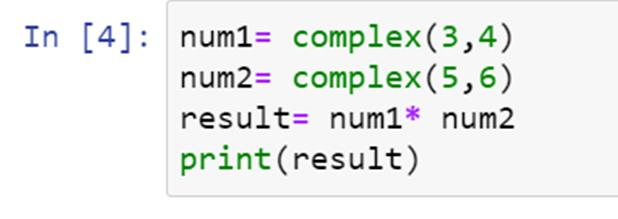We have written the complex numbers using the complex() method. The numbers are stored in variables num1 and num2, and then the multiplication is performed.

Now let’s see what the output is: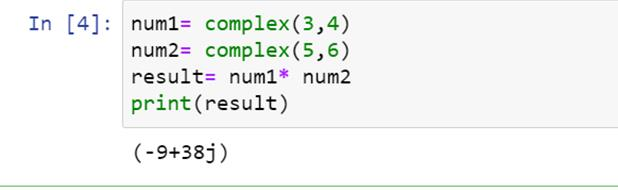We can see the output matches our calculation in the previous example.

## Multiplying String With Integers In Python

What does 'string multiplied with an integer' mean? Suppose we want to display a string(a data type of characters)  multiple times, then instead of writing it again and again, we can multiply the string by the number of times it has to be shown, and hence we can obtain the desired output.

To multiply a string with an integer in Python, we use the def()function. In the def()function, we create another function in which we mention the string variable to be repeated, followed by the number of times it has to be repeated. Then we return the multiplied value. Let's take an example here, we take the string "Hello World!" and repeat it five times.

Let's see the syntax of complete code and its output: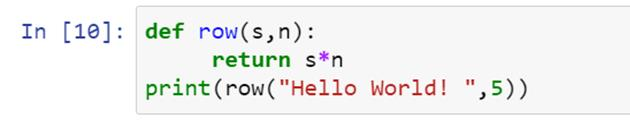We have created the row() function using the def() function. We have mentioned the string "Hello World!" as the first parameter of the row() function and then multiplication number as the second parameter.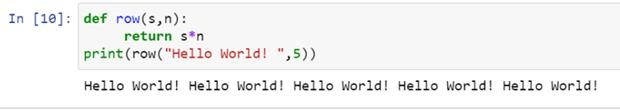We can see that the output displays the string five times.

## Use Of Functions To Multiply Numbers In Python

Functions make a program compact and easy to understand. If your program has many multiplication operations, then to save time and prevent any confusion, you can use functions to multiply numbers. The steps to define a function are as follows:

1. Declare the parameters of the function.
2. Define the operation to be performed using the function.
3. Specify the return value of the function.

We declare a function using the def keyword.

Let's see what the syntax of the code is: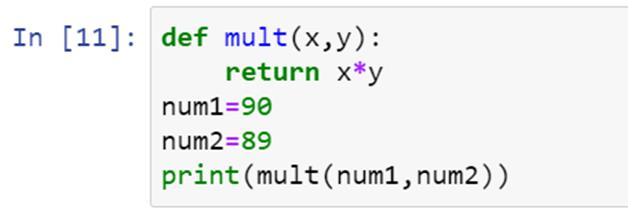Here we have defined a function mult() using the def keyword. The parameters of this function are x and y, and the operation is the multiplication of x and y, and the value returned is the product of x and y. Now let's see the output of this code: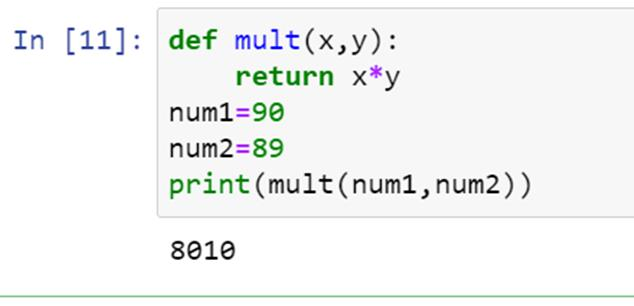We can see that the code provides the product of the entered numbers as output.

## Multiplying Lists In Python

### Multiplying One List By Another In Python

We are going to use the zip()method to multiply two lists in Python. The zip() method extracts the elements of the list. Then we multiply the elements obtained and append them into a new list. However, we have to make sure that the lists are of equal length, i.e., both the lists have an equal number of elements.

Let’s see how we write the code for this operation: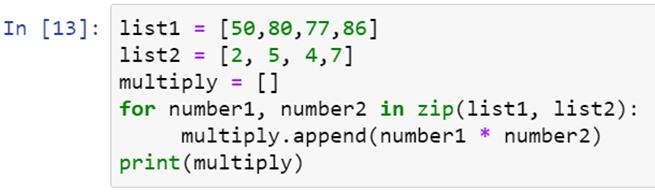Here, our two lists are 'list1' and 'list2'. Multiply is an empty list in which the compiler will append the product. Using the for loop and zip()method, we obtain the elements of each list. After multiplying the elements, we append the products in the empty list 'multiply.'

The output obtained is :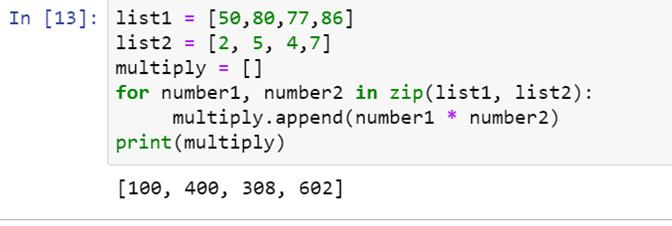We can see the products appended in the result.

### Multiplying Elements Of A List

The math module of Python has a prod() function to make our multiplication easier. We import the module first and then write the code as follows: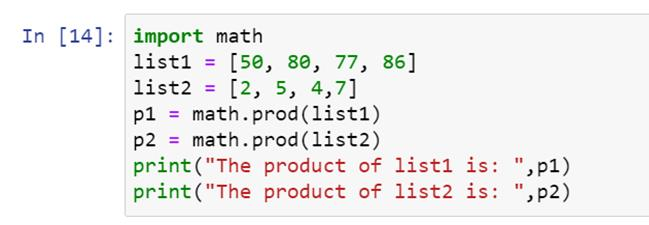We will get the product of elements of the list as output: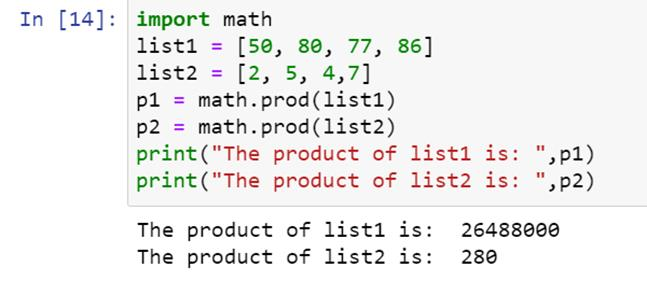### Use Of Traversal Python In Multiplying Elements Of A List

The traversal method is an iterative method to multiply elements of a list. Iterative methods comprise of looping statements. Let's see how we can use looping statements to obtain the product of list elements: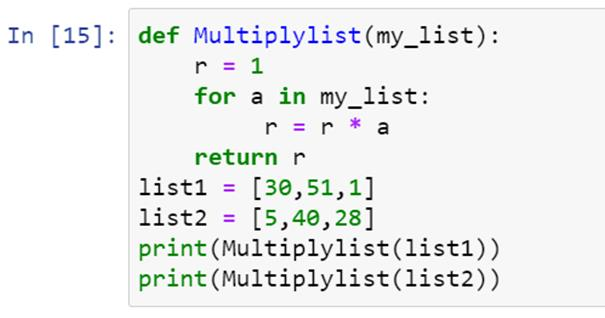Here we have defined a function Multiplylist() in which we have used a looping statement 'for.' We have initialized the variable r as one to multiply the elements one by one using the loop quickly. Finally, we have printed the product of the list. Let's see the output: